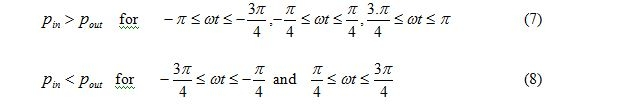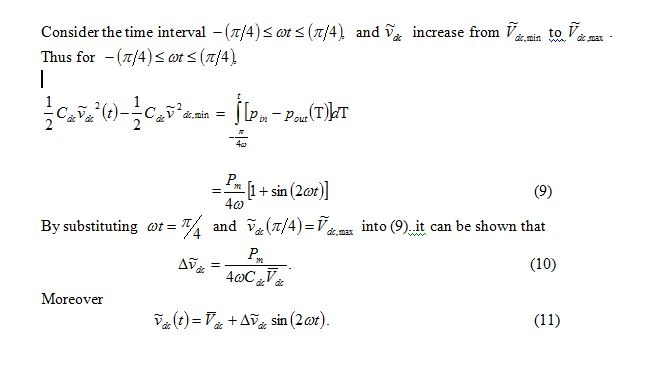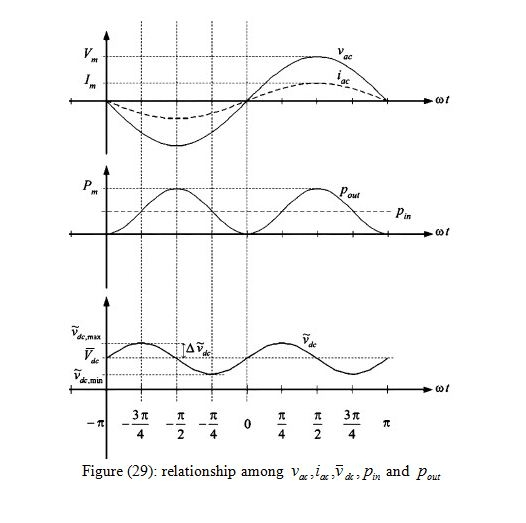Engineering

# Report on Relationship Between the Panel Power and Grid Power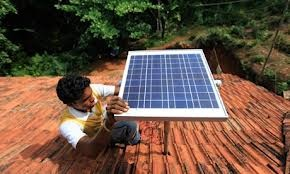The power input from the panel is  constant under a constant insolation and the power output  to the grid is time varying at twice of the grid frequency. Mathematically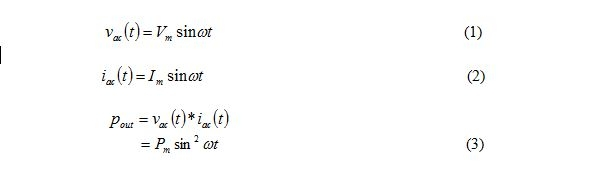Where , , and are the peak voltage, peak current and peak  angular frequency

Of the grid and respectively and  is the peak instantaneous grid power.

Fig….illustrates the relationships between    and . At the steady state, the average value of    is equal to  . That isThe capacitor  acts as a buffer for absorbing the difference between    and   . Fig. 3 depicts the low-frequency profile of the capacitor voltage varying between  and

at a dc value of   . ThusIf will increase, and vice versa. as shown in figure…